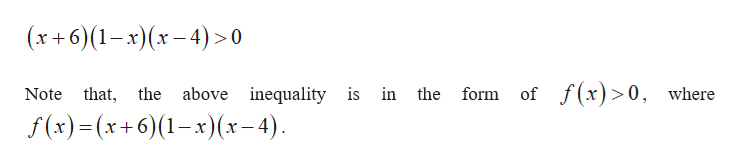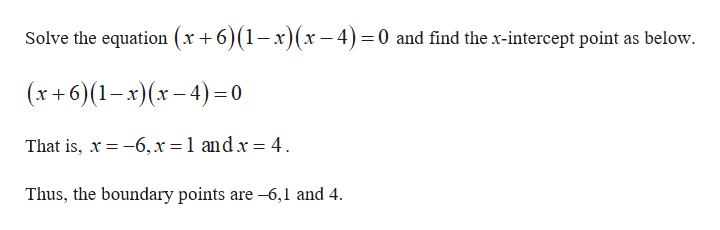O POLYNOMIAL AND RATIONAL FUNCTIONSSolving a polynomial inequality: Problem type 1Solve the following inequality.(x+6) (1-x)(x-4)>0Write your answer as an interval or union of intervals.If there is no real solution, click on "No solution"(0,D)(O,O,OOVONoSolution?X

Question

see attachmenthelp_outlineImage TranscriptioncloseO POLYNOMIAL AND RATIONAL FUNCTIONS Solving a polynomial inequality: Problem type 1 Solve the following inequality. (x+6) (1-x)(x-4)>0 Write your answer as an interval or union of intervals. If there is no real solution, click on "No solution" (0,D) (O,O,OOVO No Solution ? X fullscreen
Step 1

Solve the given ineqality and compute the solution set as follows.help_outlineImage Transcriptionclosex+6(1-x)(x-4)>0 f(x)0, where Note that, the above inequality is in the form of f (x)=(x+6)(1-x)(x-4) fullscreen
Step 2

Solve the equation f (x...help_outlineImage TranscriptioncloseSolve the equation (x +6)(1-x)(x-4) 0 and find the x-intercept point as below (x+6)(1-x)(x-4)0 That is, x 6,x = 1 andx = 4 Thus, the boundary points are-6,1 and 4 fullscreen

Want to see the full answer?

See Solution

Want to see this answer and more?

Our solutions are written by experts, many with advanced degrees, and available 24/7

See Solution
Tagged in

Other